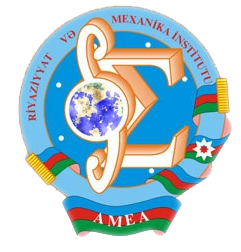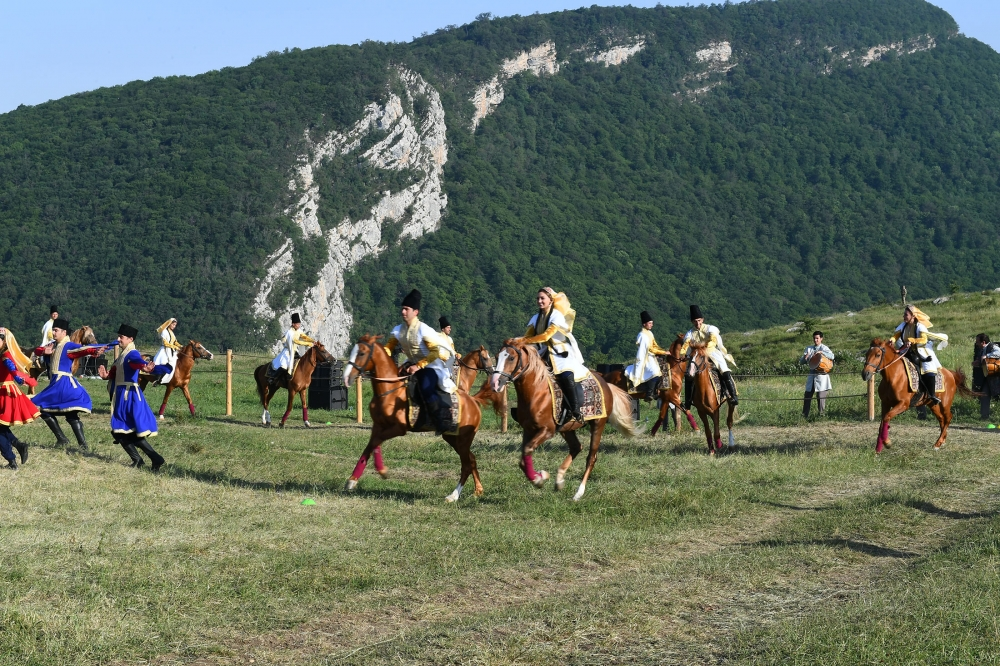Riyaziyyat və Mexanika İnstitutu

## Qarabağ xəbərləri### Şuşada Heydər Əliyev Fondunun təşkilatçılığı ilə “Musiqi irsi və Qarabağ atları Cıdır düzündə” adlı kompozisiya təqdim olunub## Transactions 2002/4/XXII

 Year: 2002 Volume: 4 Number: XXII CONTENT Academician A.D. Gadjiev [PDF] 3 MATHEMATICS Abbasov N.Yu. Abstract [PDF] On behaviour near the boundary of solutions of the second order non- uniformly degenerate parabolic equations [PDF] 7 Akhundov A.Ya. Abstract [PDF] A nonlinear parabolic inverse coefficient problem. [PDF] 19 Aliev S.A. Abstract [PDF] Limit theorems for continuous time branching processes [PDF] 25 Andriyenko V.A. Abstract [PDF] Pointwise saturation theorems for the Cezaro and Abel means of Fourier series [PDF] 31 Babaev A.M.-B. Abstract [PDF] One direct theorem in the space with the mixed norm [PDF] 40 Bilalov B.T. Abstract [PDF] On one remark on Riesz basis of sines and cosines systems [PDF] 47 Eyvazov E.H. Abstract [PDF] Refined asymptotic formulas for fundamental solutions of a higher order ordinary differential equation [PDF] 51 Gadjiev T.S. Abstract [PDF] On behaviour of solutions of nonlinear boundary value problems in conic domains [PDF] 61 Gasanov D.D. Abstract [PDF] Embedding theorems in anisotropic weight type Bn–Sobolev space [PDF] 69 Guliev V.S., Safarov Z.V. Abstract [PDF] On generalized fractional integrals, associated with the Bessel differential expansions [PDF] 75 Guliyev A.D. , Mamedov F.I. Abstract [PDF] On some qualitative properties of solutions of degenerated quasilinear equations. [PDF] 91 Hajiev F.V. Abstract [PDF] On a differentiation operation in normed vector lattices [PDF] 106 Iskenderov B.A., Sardarov V.G. Abstract [PDF] Mixed problem for Boussinesq equation in a cylindrical domain andbehavior of its solution at t > ?. [PDF] 117 Iskenderov N.Sh. Abstract [PDF] The scattering problem for a system of the first order ordinary differential equations on a semiaxis. [PDF] 135 Ismailov M.I. Abstract [PDF] The uniqueness of solution of inverse scattering problem for the system of ordinary differential equations on the whole. [PDF] 139 Khanmamedov A.Kh. Abstract [PDF] Existence of a global attractor for the plate equation in an unbounded domain [PDF] 146 Mamedov I.T. , Hassanpour S.A. Abstract [PDF] Strong solvability of the first boundary value problem for second order quasi- linear elliptic equations [PDF] 153 Mirzoyev S.S., Yagubova Kh.V. Abstract [PDF] On the completeness of one derivative chain of the root vectors of the second order operator bunches [PDF] 167 Namazov G.K., Mehraliyev Ya.T. Abstract [PDF] Investigation of a classical solution of a non-self- adjoint one-dimensional inverse boundary value problem for a higher order differential equation [PDF] 174 Novruzov E.B. Abstract [PDF] On existence of absorbing set on initial boundary value problem for a non-linear degenerate equation [PDF] 185 Shahbazov A.I., Dehghan Ya.N. Abstract [PDF] Weighted composition operators on the spaces of vector-valued functions [PDF] 193 MECHANICS Akhmedov N.K., Shirinov T.V. Abstract [PDF] Asymptotic analysis of a space problem of elasticity theory for nonhomogeneous hollow cone of small thickness [PDF] 197 Gadjiev V.D., Akhadova S.R. Abstract [PDF] Approximate – analytical solution of stability problem of nonhomogeneous elastic rectangular plate lying on nonhomogeneous base [PDF] 204 Hajiyev V.J., Mirsalimov V.M. Abstract [PDF] Limit-equilibrium state of bush of contact pair in the presence of cracks with yielded end areas [PDF] 209 Latifov F.S. Abstract [PDF] Asymptotic estimations of eigen-frequencies of vibrations of stifenfed constructions, filled with liquid [PDF] 219 Mehdiyev M.F., Mardanov I.D., Amrahova A.R. Abstract [PDF] Asymptotic analysis of bending problem for transversal-isotropic plate of variable thickness [PDF] 223 Rzayev O.G. Abstract [PDF] On the buckling of the composite circular plate with two parallel penny-shaped cracks [PDF] 237 Talybly L.Kh. Abstract [PDF] On modelling problem of delayed fracture processes of constructions [PDF] 247 Yusifov M.O. Abstract [PDF] The transverse vibration of a pile in visco-elastic medium Applied Problems of Mathematics and Mechanics [PDF] 253 Akhundov M.B. Abstract [PDF] Durability criterion of fibrous composites [PDF] 257 S.Yu. Guliyeva. Abstract [PDF] Fatigue failure of submerged hollow thin-walled with fixed ends cylindrical element from airy naves action [PDF] 262
Azərbaycanda COVID-19 ilə bağlı statistika
• Virusa yoluxan

596973

• Sağalan

565521

• Yeni yoluxan

0

• Aktiv xəstə

23469

• Ölüm halı

7983

• Test edilib

5,608,158